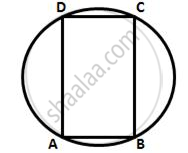Share

Prove That: the Parallelogram, Inscribed in a Circle, is a Rectangle. - ICSE Class 10 - Mathematics

ConceptArc and Chord Properties - Angle in a Semi-circle is a Right Angle

Question

Prove that:
the parallelogram, inscribed in a circle, is a rectangle.

SolutionLet ABCD be a parallelogram, inscribe in a circle,
Now, ∠BAD = ∠BCD
(Opposite angles of a parallelogram are equal)
And ∠BAD = ∠BCD = 180°
(pair of opposite angles in a cyclic quadrilateral are supplementary)
∠BAD = ∠BCD = (180°) /2= 90°
∥y, the other two angles are 90 and opposite pair of sides
Are equal.
∴ ABCD is a rectangle.

Is there an error in this question or solution?

Video TutorialsVIEW ALL 

Solution Prove That: the Parallelogram, Inscribed in a Circle, is a Rectangle. Concept: Arc and Chord Properties - Angle in a Semi-circle is a Right Angle.
S